# Texas Go Math Grade 3 Lesson 8.4 Answer Key Multiply with 8

Refer to our Texas Go Math Grade 3 Answer Key Pdf to score good marks in the exams. Test yourself by practicing the problems from Texas Go Math Grade 3 Lesson 8.4 Answer Key Multiply with 8.

## Texas Go Math Grade 3 Lesson 8.4 Answer Key Multiply with 8

Essential Question
What strategies can you use to multiply with 8?

Unlock the Problem
A scorpion has 8 legs. How many legs do 5 scorpions have?

• How many legs does one scorpion have?
8 legs one scorpion have.

Explanation:
Number of legs one scorpion have = 8.

• What are you asked to find?
How many legs do 5 scorpions have?

Explanation:
A scorpion has 8 legs. How many legs do 5 scorpions have?

Find 5 × 8.
One Way
Use doubles.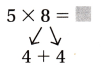Think: The factor 8 is an even number. 4 + 4 = 8
5 × 4 = ___
20 doubled is __.
5 × 8 = __
So, 5 scorpions have ___ legs.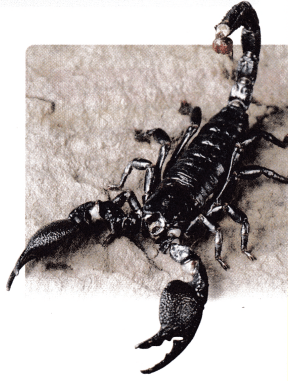Another Way
Use a number line.

Use the number line to show 5 jumps of 8.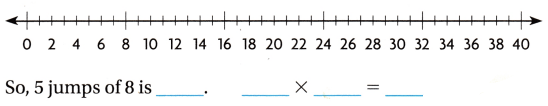• Describe two different ways you can use doubles to find 6 × 8.

Be sure to count the spaces between the tick marks, not the tick marks.

Example
Use the Associative Property of Multiplication.
Scorpions have two eyes on the top of the head, and usually two to five pairs along the front corners of the head. If each scorpion has 6 eyes, how many eyes would 8 scorpions have?Math Talk
Mathematical Processes
When you multiply with 8, will the product always be even? Explain.

Share and Show
Question 1.
Explain one way you can find 4 × 8.
4 × 8 = (4 × 4) × 2 = 32.

Explanation:
4 × 8 = 4 × (4 × 2)
= (4 × 4) × 2
= 16 × 2
= 16 + 16
= 32.

Find the product.

Question 2.
3 × 8 = __
3 × 8 = 24.

Explanation:
3 × 8 = 3 × (4 × 2)
= (3 × 4) × 2
= 12 × 2
= 12 + 12
= 24.

__ = 8 × 2
8 × 2 = 16.

Explanation:
8 × 2 = (4 × 2) × 2
= 4 × (2 × 2)
= 4 × 4
= 16.

Question 4.
__ = 7 × 8
7 × 8 = 56.

Explanation:
7 × 8 = 7 × (4 × 2)
= (7 × 4) × 2
= 28 × 2
= 28 + 28
= 56.

Question 5.
9 × 8 = __
9 × 8 = 72.

Explanation:
9 × 8 = 9 × (4 × 2)
= (9 × 4) × 2
= 36 × 2
= 36 + 36
= 72.

Question 6.
__ = 6 × 8
6 × 8 = 48.

Explanation:
6 × 8 = 6 × (4 × 2)
= (6 × 4) × 2
= 24 × 2
= 24 + 24
= 48.

Question 7.
10 × 8 = __
10 × 8 = 80.

Explanation:
10 × 8 = 10 × (4 × 2)
= (10 × 4) × 2
= 40 × 2
= 40 + 40
= 80.

Question 8.
___ = 8 × 3
8 × 3 = 24.

Explanation:
8 × 3 = (2 × 4) × 3
= (3 × 4) × 2
= 12 × 2
= 12 + 12
= 24.

0 × 8 = ___
0 × 8 = 0.

Explanation:
0 × 8 = 0 × (4 × 2)
= (0 × 4) × 2
= 0 × 2
= 0 + 0
= 0.

Problem Solving
H.O.T. Algebra Complete the table.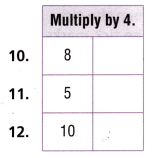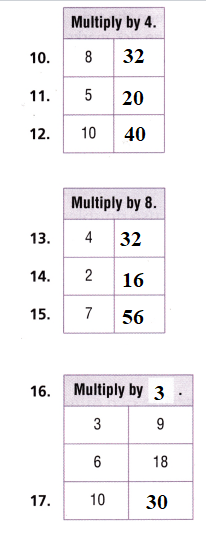Explanation:
Multiply by 4:
10. 8 × 4 = 32.
11. 5 × 4 = 20.
12. 10 × 4 = 40.
Multiply by 8:
13. 4 × 8 = 4 × (4 × 2)
= (4 × 4) × 2
= 16 × 2
= 16 + 16
= 32.
14. 2 × 8 = 2 × (4 × 2)
= 2 × 8
= 8 + 8
= 16.
15. 7 × 8 = 7 × (4 × 2)
= (7 × 4) × 2
= 28 × 2
= 28 + 28
= 56.
16. Multiply by ??:
3 × ?? = 9
=> ?? = 9 ÷ 3
=> ?? = 3.
6 × 3 = 18.
17. 10 × 3 = 30.

Problem Solving
Use the table for 18-21.
Question 18.
About how much rain falls in the Chihuahuan Desert in 6 years? Explain how you can use doubles to find the answer.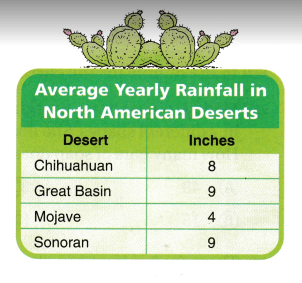Number of inches of rainfall in Chihuahuan Desert in a 6 years = 48.

Explanation:
Number of inches of rainfall in Chihuahuan Desert in a year = 8.
Number of inches of rainfall in Chihuahuan Desert in a 6 years = 6 × Number of inches of rainfall in Chihuahuan Desert in a year
= 6 × 8
= 6 × (4 × 2)
= (6 × 4) × 2
= 24 × 2
= 24 + 24
= 48.

Multi-Step Predict In 2 years, about how many more inches of rain will fall in the Sonoran Desert than in the Chihuahuan Desert? Explain.
2 more inches of rain will fall in the Sonoran Desert than in the Chihuahuan Desert.

Explanation:
Number of inches of rainfall in the Sonoran Desert in a year = 9.
Number of inches of rainfall in Sonoran Desert in 2 years = 2 × Number of inches of rainfall in Sonoran Desert in a year
= 2 × 9
= 9 + 9
= 18.
Number of inches of rainfall in the Chihuahuan Desert in a year = 8.
Number of inches of rainfall in Chihuahuan Desert in 2 years = 2 × Number of inches of rainfall in Chihuahuan Desert in a year
= 2 × 8
= 2 × (4 × 2)
= (2× 4) × 2
= 8 × 2
= 8 + 8
= 16.
Difference:
Number of inches of rainfall in Sonoran Desert in a 2 years – Number of inches of rainfall in Chihuahuan Desert in a 2 years
= 18 – 16
= 2.Question 20.
Write Math Pose a Problem Look back at Problem 19. Write and show how to solve a similar problem by comparing two different deserts.
Question:
Predict In 2 years, about how many more inches of rain will fall in the great basin Desert than in the Mojave Desert? Explain.
Solution:
10 more inches of rain will fall in the great basin Desert than in the Mojave Desert.

Explanation:
Number of inches of rainfall in great basin Desert in a year = 9.
Number of inches of rainfall in great basin Desert in a 2 years = 2 × Number of inches of rainfall in great basin Desert in a year
= 2 × 9
= 9 + 9
= 18.
Number of inches of rainfall in Mojave Desert in a year = 4.
Number of inches of rainfall in Mojave Desert in a 2 years = 2 × Number of inches of rainfall in Mojave Desert in a year
= 2 × 4
= 4 + 4
= 8.
Difference:
Number of inches of rainfall in great basin Desert in a 2 years – Number of inches of rainfall in Mojave Desert in a 2 years
= 18 – 8
= 10.

Question 21.
H.O.T. What strategy can you use to find about how many inches of rain will fall in the Mohave Desert in 20 years?The Associative Property of Multiplication strategy can be used to find about how many inches of rain will fall in the Mohave Desert in 20 years.
Number of inches of rainfall in Mojave Desert in a 20 years = 80.

Explanation:
Number of inches of rainfall in Mojave Desert in a year = 4.
Number of inches of rainfall in Mojave Desert in a 20 years = 20 × Number of inches of rainfall in Mojave Desert in a year
= 20 × 4
= (2 × 10) × 4
= 2 × (10 × 4)
= 2 × 40
= 40 + 40
= 80.

Scorpions have 8 legs for walking and 2 long, claw-like pincers used to hold their food. What multiplication sentences can help you find how many pincers and legs 8 scorpions have?
Total number of pincers and legs 8 scorpions have = 10.

Explanation:
Number of legs for walking Scorpions have = 8.
Number of long claw-like pincers Scorpions have = 2.
Total number of pincers and legs 8 scorpions have = Number of legs for walking Scorpions have + Number of long claw-like pincers Scorpions have
= 8 + 2
= 10.

Fill in the bubble for the correct answer choice.
Question 23.
Maddy is inventing a new kind of soap. Her soaps come in 7 different shapes. She makes 8 bars in each shape. How many bars of soap does Maddy make?
(A) 49
(B) 15
(C) 56
(D) 64
Number of bars of soap Maddy makes = 56.
(C) 56.

Explanation:
The number of different shapes Her soaps came = 7.
Number of bars she makes in each shape = 8.
Number of bars of soap Maddy makes = Number of different shapes Her soaps came × Number of bars she makes in each shape
= 7 × 8
= 7 × (4 × 2)
= (7 × 4) × 2
= 28 × 2
= 28 + 28
= 56.

Question 24.
There are 3 spiders in a glass case. Each spider has 8 legs. How many legs do the 3 spiders have?
(A) 24
(B) 18
(C) 38
(D) 9
Number of legs the 3 spiders have = 24.
(A) 24.

Explanation:
Number of spiders in a glass case = 3.
Number of legs each spider has = 8.
Number of legs the 3 spiders have = Number of spiders in a glass case × Number of legs each spider has
= 3 × 8
= 3 × (4 × 2)
= (3 × 4) × 2
= 12 × 2
= 12 + 12
= 24.

Question 25.
Multi-Step The table shows the number of markers in each pack. Ms. Wilson buys 5 packs of bright markers and 5 packs of glitter markers. How many more bright markers does she buy than glitter markers?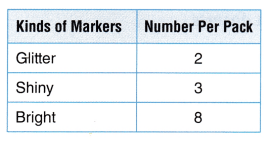(A) 50
(B) 30
(C) 40
(D) 10
30 more bright markers she buys than glitter markers.
(B) 30.

Explanation:
Number of packs of bright markers Ms. Wilson buys = 5.
Number of bright markers in a pack = 8.
Total number of bright markers in all packs Ms. Wilson buys = Number of packs of bright markers Ms. Wilson buys × Number of bright markers in a pack
= 5 × 8
= 5 × (4 × 2)
= (5 × 4) × 2
= 20 × 2
= 20 + 20
= 40.
Number of packs of glitter markers Ms. Wilson buys = 5.
Number of glitter markers  in a pack = 2.
Total number of glitter markers in all packs Ms. Wilson buys = Number of packs of glitter markers Ms. Wilson buys × Number of glitter markers in a pack
= 5 × 2
= 5 + 5
= 10.
Difference:
Total number of bright markers in all packs Ms. Wilson buys – Total number of glitter markers in all packs Ms. Wilson buys
= 40 – 10
= 30.

Texas Test Prep
Question 26.
A black widow spider has 8 legs. How many legs do 4 black widow spiders have?
(A) 12
(B) 24
(C) 4
(D) 32
Number of legs 4 black widow spiders have = 32.
(D) 32.

Explanation:
Number of legs a black widow spider has = 8.
Number of  black widow spider = 4.
Number of legs 4 black widow spiders have = Number of legs a black widow spider has × Number of  black widow spider
= 8 × 4
= (2 × 4) × 4
= 2 × (4 × 4)
= 2 × 16
= 16 + 16
= 32.

### Texas Go Math Grade 3 Lesson 8.4 Homework and Practice Answer Key

Complete the table.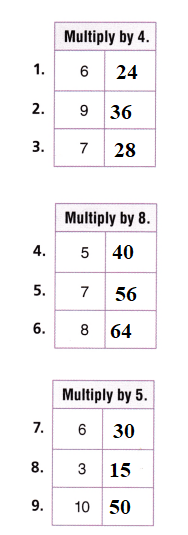Explanation:
Multiply by 4:
1. 6 × 4 = 6 × (2 × 2)
= (6 × 2) × 2
= 12 × 2
= 12 + 12
= 24.
2. 9 × 4 = 9 × (2 × 2)
= (9 × 2) × 2
= 18 × 2
= 18 + 18
= 36.
3. 7 × 4 = 7 × (2 × 2)
= (7 × 2) × 2
= 14 × 2
= 14 + 14
= 28.
Multiply by 8:
5. 5 × 8 = 5 × (4 × 2)
= (5 × 4) × 2
= 20 × 2
= 20 + 20
= 40.
6. 7 × 8 = 7 × (4 × 2)
= (7 × 4) × 2
= 28 × 2
= 28 + 28
= 56.
7. 8 × 8 = 8 × (4 × 2)
= (8 × 4) × 2
= 32 × 2
= 32 + 32
= 64.
Multiply by 5:
8. 6 × 5 = (2 × 3) × 5
= 2 × (3 × 5)
= 2 × 15
= 15 + 15
= 30.
9. 3 × 5 = 15.
10. 10 × 5 = (2 × 5) × 5
= 2 × (5 × 5)
= 2 × 25
= 25 + 25
= 50.

Problem Solving
Use the table for 10-12.
Question 10.
Jill is making 4 batches of punch. Write multiplication sentences to show how many total cups of strawberries and crushed ice Jill needs.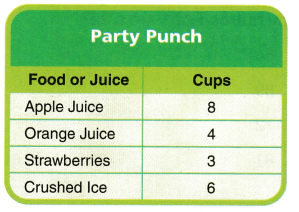Total number of cups of strawberries and crushed ice Jill needs = 36.

Explanation:
Number of batches of punch Jill is making = 4.
Number of cups of strawberries = 3.
Number of cups of crushed ice = 6.
Total number of cups of strawberries and crushed ice Jill needs = Number of batches of punch Jill is making (Number of cups of strawberries + Number of cups of crushed ice)
= 4 × (3 + 6)
= 4 × 9
= 36.

Go Math Practice and Homework Lesson 8.4 Answer Key Question 11.
How many cups of apple juice will Jill need to make 8 batches of punch? Explain how you can use doubles to find the answer.
Number of cups of apple juice Jill needs to make 8 batches of punch = 64.

Explanation:
Number of cups of apple juice = 8.
Number of batches of punch Jill is making = 8.
Number of cups of apple juice Jill needs to make 8 batches of punch = Number of cups of apple juice × Number of batches of punch Jill is making
= 8 × 8
= 8 × (4 × 2)
= (8 × 4) × 2
= 32 × 2
= 32 + 32
= 64.

Question 12.
Webb wants to make 7 batches of punch. What strategy can you use to find how many cups of crushed ice he will need?
The Associative Property of Multiplication strategy can be used to find how many cups of crushed ice he needs.
Number of cups of crushed ice Jill needs to make 7 batches of punch = 42.

Explanation:
Number of cups of crushed ice = 6.
Number of batches of punch Jill is making = 7.
Number of cups of crushed ice Jill needs to make 7 batches of punch = Number of cups of crushed ice × Number of batches of punch Jill is making
= 6 × 7
= (2 × 3) × 7
= 2 × (3 × 7)
= 2 × 21
= 21 + 21
= 42.

Texas Test Prep
Lesson Check
Question 13.
A florist is making 8 bouquets of flowers. She needs 7 roses for each bouquet. How many roses does the florist need?
(A) 64
(B) 48
(C) 56
(D) 28
Number of roses the florist needs = 56.
(C) 56.

Explanation:
Number of roses for each bouquet she needs = 7.
Number of bouquets of flowers a florist is making = 8.
Number of roses the florist needs = Number of roses for each bouquet she needs × Number of bouquets of flowers a florist is making
= 7 × 8
= 7 × (4 × 2)
= (7 × 4) × 2
= 28 × 2
= 28 + 28
= 56.

Question 14.
Elena has a bookshelf with 4 shelves. There are 8 books on each shelf. How many books does Elena have?
(A) 48
(B) 32
(C) 40
(D) 36
Number of books Elena have = 32.
(B) 32.

Explanation:
Number of shelves Elena has a bookshelf = 4.
Number of books on each shelf = 8.
Number of books Elena have = Number of shelves Elena has a bookshelf × Number of books on each shelf
= 4 × 8
= 4 × (4 × 2)
= (4 × 4) × 2
= 16 × 2
= 16 + 16
= 32.

Question 15.
A toy store sells train sets. Each set has 9 railroad cars in it. If the store sells 8 train sets in one day, how many railroad cars are sold?
(A) 64
(B) 89
(C) 72
(D) 81
Number of railroad cars are sold = 72.
(C) 72.

Explanation:
Number of railroad cars each set has = 9.
Number of train sets in one day the store sells = 8.
Number of railroad cars are sold = Number of railroad cars each set has × Number of train sets in one day the store sells
= 9 × 8
= 9 × (4 × 2)
= (9 × 4) × 2
= 36 × 2
= 36 + 36
= 72.

Question 16.
A chef is making 5 batches of potato salad. He needs 8 cups of dressing for each salad. How many cups of dressing does he need?
(A) 58 cups
(B) 45 cups
(C) 40 cups
(D) 13 cups
Number of cups of dressing does he needs = 40.
(C) 40 cups.

Explanation:
Number of batches of potato salad a chef is making = 5.
Number of cups of dressing for each salad he needs = 8.
Number of cups of dressing does he needs = Number of batches of potato salad a chef is making × Number of cups of dressing for each salad he needs
= 5 × 8
= 5 × (4 × 2)
= (5 × 4) × 2
= 20 × 2
= 20 + 20
= 40.

Multi-Step Giselle buys 3 packs of baseball cards and 6 packs of soccer cards. Each pack has 8 cards in it. How many cards does Giselle buy?
(A) 18
(B) 48
(C) 24
(D) 72
Number of cards Giselle buys = 72.
(D) 72.

Explanation:
Number of packs of baseball cards Giselle buys = 3.
Number of packs of soccer cards Giselle buys = 6.
Number of cards each pack has = 8.
Number of cards Giselle buys = (Number of packs of baseball cards Giselle buys + Number of packs of soccer cards Giselle buys) × Number of cards each pack has
= (3 + 6) × 8
= 9 × (4 × 2)
= (9 × 4) × 2
= 36 × 2
= 36 + 36
= 72.

Question 18.
Multi-Step Jamal buys 4 sets of animal postcards and 5 sets of nature postcards. Each set has 6 cards. How many postcards does Jamal buy?
(A) 30
(B) 56
(C) 54
(D) 64
Number of postcards Jamal buys = 54.
(C) 54.

Explanation:
Number of sets of animal postcards Jamal buys = 4.
Number of sets of nature postcards Jamal buys = 5.
Number of cards in each set = 6.
Number of postcards Jamal buys = (Number of sets of animal postcards Jamal buys + Number of sets of nature postcards Jamal buys) × Number of cards in each set
= (4 + 5) × 6
= 9 × (3 × 2)
= (9 × 3) × 2
= 27 × 2
= 27 + 27
= 54.

Scroll to Top